# Boundary Conditions

## Introduction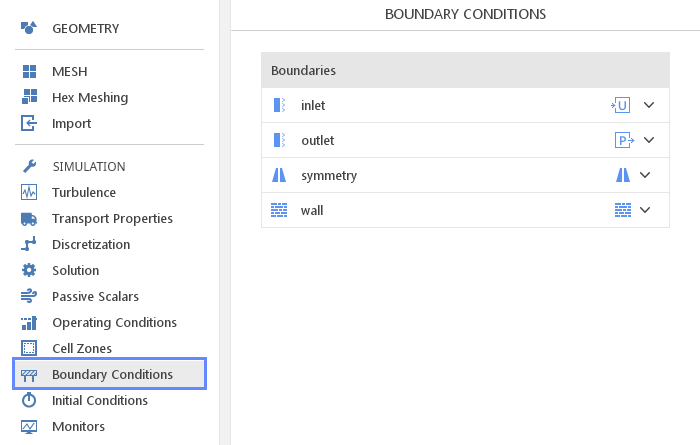The panel defines boundary conditions for each boundary of the numerical mesh. Most of the boundary conditions are reasonably specified by default, and only minor changes might be required to adjust in a given case.

## Boundary Conditions List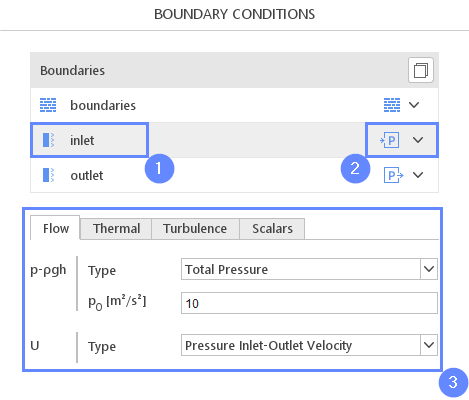The Boundaries list displays all boundaries sorted alphabetically 1. On the right-hand side of each boundary name the type of mesh boundary is shown 2. The list of available boundary types can be displayed by pressing the arrow next to the icon. Selection of a given boundary opens a panel with detailed options below the boundary list 3.

## Boundary Condition Character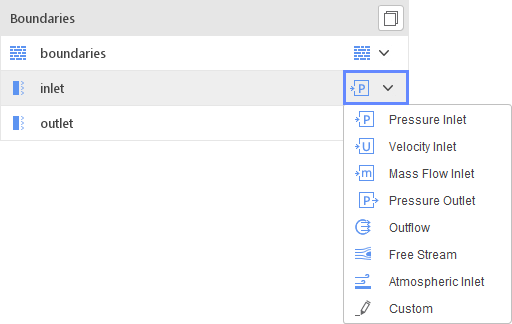Boundary condition character is a set of predefined boundary conditions for each of the fields. The list of available characters depends on the boundary type and the currently selected solver.

## Boundary Condition Groups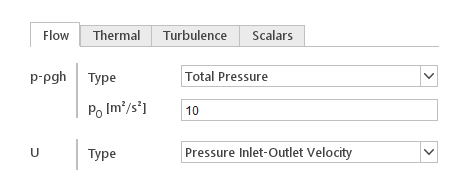Boundary condition options for related fields are grouped and placed under one tab.

Groups:

• Flow - velocity and pressure
• Thermal - temperature and radiation fields
• Turbulence - turbulence fields
• Phases - phases in multi-phase simulation
• Species - species fields in combustion simulation
• Scalars - additional passive scalar fields created by the user

## Boundary Condition Types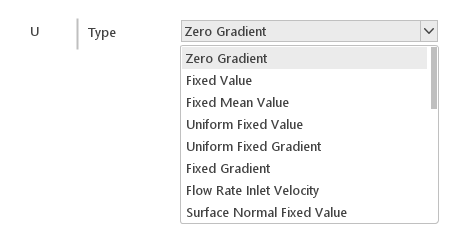The definition of a boundary condition includes the type and optional input required by a particular type of the boundary condition.

Boundary condition types and inputs:

General Boundary Conditions

• Fixed Value - value of a field is fixed on the boundary (Dirichlet boundary condition) $$\phi = const$$
Value - value of the field
• Uniform Fixed Value - uniform value of a field on the boundary that can change in time $$\phi=f(t)$$
Value - time dependent value of the field
• Fixed Gradient - surface normal derivative of a field is fixed on the boundary (Neumann boundary condition) $$\nabla \phi \cdot \vec{n} = const$$
Gradient - value of the surface normal derivative on the boundary
• Uniform Fixed Gradient - surface normal derivative of a field that can change in time $$\nabla \phi \cdot \vec{n} = f(t)$$
Gradient - time dependent derivative of the field
• Zero Gradient - derivative of a field normal to the boundary is equal to zero (this is a special case of Neumann boundary condition) $$\nabla \phi \cdot \vec{n} = 0$$
• Symmetry - enforces a symmetry constraint - all vectors are tangent to the boundary and gradients normal to the boundary are zero
• Inlet-Outlet - specifies a generic outflow conditions. Mixed boundary condition behaves like Zero Gradient when the flow is out of the domain and like Fixed Value when there is backward flow into the domain
Inlet Value - value at the boundary in case of backward flow
• Uniform Inlet-Outlet - similar to Inlet-Outlet but inlet value can change in time
Inlet Value - value at the boundary in case of backward flow
• Free Stream - specifies free-stream value, behaves similar to Inlet-Outlet
Freestream Value - value in the free-stream
• Turbulent Inlet - generates a fluctuating inlet condition by adding a random component to a reference (mean) field
Reference Field - mean value of the field
Fluctuation Scale - fluctuation scale
• Mapped Inlet - sets inlet value to the average value on another boundary
Map From - boundary to calculate inlet value

Temperature Boundary Conditions

• Total Temperature - fixes total temperature at the inlet
$$T_{0}$$ - stagnation temperature
• Inlet-Outlet Total Temperature - similar to Total Temperature but behaves like Zero Gradient in case of backward flow
$$T_{0}$$ - stagnation temperature
• Heat Flux - fixes heat flux at the boundary
q - heat flux at the boundary
• Wall Heat Transfer - convective heat transfer boundary condition
$$T_{\infty}$$ - ambient temperature
h - heat transfer coefficient
• Power - fixes heat transfer power at the boundary
q - power through the boundary

Velocity Boundary Conditions

• Surface Normal Fixed Value - specifies vector normal to the boundary with a given value
Reference Value - normal vector (positive means into the domain)
• Pressure Inlet-Outlet Velocity - similar to Zero Gradient but corrects inflow velocity on boundaries where pressure is specified
• Pressure Normal Inlet-Outlet Velocity - similar to Pressure Inlet-Outlet Velocity but forces inflow velocity to be normal to the boundary
• Pressure Directed Inlet-Outlet Velocity - similar to Pressure Inlet-Outlet Velocity but forces inflow velocity to be in a specified direction
Direction - direction of the inflow velocity
• Flow Rate Inlet Velocity - fixes volumetric or mass flow rate at the boundary
Flow Rate - volumetric or mass flow rate
• Slip - slip constraint - forces velocity to be tangent to the boundary
• No-Slip - fixes the velocity to zero at walls
• Fixed Normal Slip - sets the boundary-normal component from a fixed value
Value - boundary-normal component
• Cylindrical Inlet - specifies velocity in a cylindrical coordinate system
Centre - centre of the coordinate system
Axis - axis of the coordinate system
Axial Velocity - axial velocity
RPM - rotational speed used to define tangential velocity
• Moving Wall Velocity - condition for cases with moving walls
Value - velocity of the wall
• Rotating Wall Velocity - condition for cases with rotating walls
Origin - centre of the coordinate system
Axis - axis of the coordinate system
$$omega$$ - rotational speed [rad/s]

Pressure Boundary Conditions

• Total Pressure - fixes total pressure at the boundary
$$p_{0}$$ - total pressure
• Uniform Total Pressure - similar to Total Pressure but can change total pressure with time
$$p_{0}$$ - total pressure as a function of time
• Fixed Flux Pressure - sets the pressure gradient such that the flux on the boundary is that specified by the velocity boundary condition
• Hydrostatic Pressure - static pressure condition for pseudo hydrostatic pressure
• Hydrostatic Total Pressure - total pressure condition for pseudo hydrostatic pressure
$$p_{0}$$ - total pressure
• Phase Hydrostatic Pressure - phase-based hydrostatic pressure condition
• Wave Transmissive - wave transmissive outflow condition
• Fan - pressure inlet or outlet total pressure condition for a fan
$$p_{0}$$ - external total pressure
Direction - direction of the fan flow (in/out)
File Name - path to the file with fan curve (OpenFOAM format)
Out Of Bounds - what to do when flow goes outside the fan curve data

Turbulence Boundary Conditions

• Turbulent Intensity Inlet - turbulent kinetic energy is calculated from velocity and specified intensity
Intensity - turbulent intensity level
• Turbulent Mixing Length Inlet - turbulent frequency and dissipation rate are calculated from mixing length and turbulent kinetic energy ($$\epsilon = \frac{C_{\mu}^{0.75} k^{1.5}}{ L_{\omega}} = k^{0.5} C_\mu^{0.25} L$$)
Mixing Length - mixing length

Multiphase Boundary Conditions

• Variable Height Inlet - velocity boundary condition for multiphase flow based on a user-specified volumetric flow rate. The flow rate is made proportional to the phase fraction alpha at each face
Flow Rate - phase volumetric flow rate
• Variable Height - phase fraction condition based on the local flow conditions, whereby the values are constrained to lay between user-specified upper and lower bounds
Lower Bound - lower bound
Upper Bound - upper bound
• Outlet Phase Mean Velocity - adjusts the velocity for the given phase to achieve the specified mean
$$U_{mean}$$ - mean phase velocity
• Interstitial Inlet Velocity - inlet velocity in which the actual interstitial velocity is calculated by dividing the specified velocity field with the local phase-fraction
Velocity - interstitial velocity
• Constant Contact Angle - fixes equilibrium contact angle
$$\theta_{0}$$ - equilibrium contact angle

• Marshak - Marshak boundary condition for incident radiation
• Marshak Fixed Temperature - Marshak boundary condition for incident radiation with specified temperature
$$T_{rad}$$ - radiation temperature

Coupled Boundary Condition

• Cyclic (AMI/ACMI) - exchanges information between cyclic (AMI/ACMI) boundaries
• Fixed Jump (AMI) - specifies offset of field value between two cyclic (AMI) boundaries
Jump - field offset between two boundaries
• Uniform Jump (AMI) - specifies time varying offset of field value between two cyclic (AMI) boundaries
Jump Table - field offset function between two boundaries
• Coupled Temperature - couples temperature and heat flux between two mesh regions
Resistance - enable or disable additional resistance layer between regions
$$\delta$$ - thickness of the resistance layer
$$\kappa$$ - thermal conductivity of the resistance layer
• Coupled Temperature and Radiation - similar to Coupled Temperature but includes radiation in the heat flux balance

Special Boundary Conditions

• Atmospheric Inlet - calculates velocity and turbulence distributions from atmospheric boundary layer model
Flow Dir. - direction of the air flow
Vertical Dir. - vertical direction (perpendicular to the ground)
$$U_{ref}$$ - reference velocity
$$z_{ref}$$ - reference height
$$z_{0}$$ - roughness height
$$z_{ground}$$ - ground location